# Decagon

Regular decagon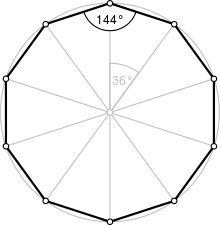A regular decagon
Type Regular polygon
Edges and vertices 10
Schläfli symbol {10}, t{5}
Coxeter diagram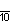Symmetry group Dihedral (D10), order 2×10
Internal angle (degrees) 144°
Dual polygon Self
Properties Convex, cyclic, equilateral, isogonal, isotoxal

In geometry, a decagon is a ten-sided polygon or 10-gon.

## Regular decagon

A regular decagon has all sides of equal length and each internal angle will always be equal to 144°. Its Schläfli symbol is {10}  and can also be constructed as a truncated pentagon, t{5}, a quasiregular decagon alternating two types of edges.

### Area

The area of a regular decagon of side length a is given by:In terms of the apothem r (see also inscribed figure), the area is:In terms of the circumradius R, the area is:An alternative formula iswhere d is the distance between parallel sides, or the height when the decagon stands on one side as base, or the diameter of the decagon's inscribed circle. By simple trigonometry,and it can be written algebraically as### Sides

The side of a regular decagon inscribed in a unit circle is, where ϕ is the golden ratio,.

### Construction

As 10 = 2 × 5, a power of two times a Fermat prime, it follows that a regular decagon is constructible using compass and straightedge, or by an edge-bisection of a regular pentagon.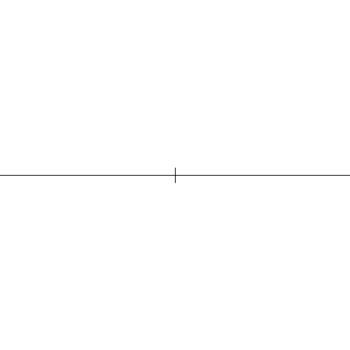Construction of decagon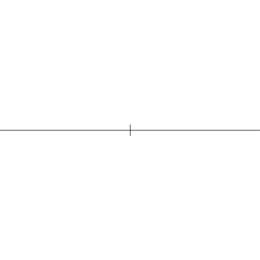Construction of pentagon

An alternative (but similar) method is as follows:

1. Construct a pentagon in a circle by one of the methods shown in constructing a pentagon.
2. Extend a line from each vertex of the pentagon through the center of the circle to the opposite side of that same circle. Where each line cuts the circle is a vertex of the decagon.
3. The five corners of the pentagon constitute alternate corners of the decagon. Join these points to the adjacent new points to form the decagon.

## The golden ratio in decagon

Both in the construction with given circumcircle as well as with given side length is the golden ratio dividing a line segment by exterior division the determining construction element.

• In the construction with given circumcircle the circular arc around G with radius GE3 produces the segment AH, whose division corresponds to the golden ratio.• In the construction with given side length the circular arc around D with radius DA produces the segment E10F, whose division corresponds to the golden ratio.Decagon with given circumcircle, animation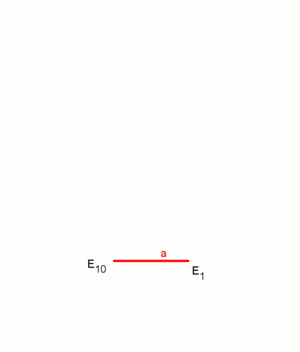Decagon with a given side length, animation

## Symmetry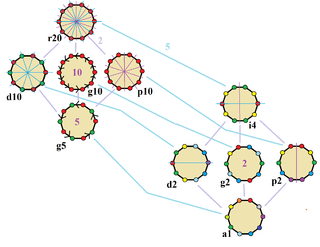Symmetries of a regular decagon. Vertices are colored by their symmetry positions. Blue mirrors are drawn through vertices, and purple mirrors are drawn through edge. Gyration orders are given in the center.

The regular decagon has Dih10 symmetry, order 20. There are 3 subgroup dihedral symmetries: Dih5, Dih2, and Dih1, and 4 cyclic group symmetries: Z10, Z5, Z2, and Z1.

These 8 symmetries can be seen in 10 distinct symmetries on the decagon, a larger number because the lines of reflections can either pass through vertices or edges. John Conway labels these by a letter and group order. Full symmetry of the regular form is r20 and no symmetry is labeled a1. The dihedral symmetries are divided depending on whether they pass through vertices (d for diagonal) or edges (p for perpendiculars), and i when reflection lines path through both edges and vertices. Cyclic symmetries in the middle column are labeled as g for their central gyration orders.

Each subgroup symmetry allows one or more degrees of freedom for irregular forms. Only the g10 subgroup has no degrees of freedom but can seen as directed edges.

The highest symmetry irregular decagons are d10, a isogonal decagon constructed by five mirrors which can alternate long and short edges, and p10, an isotoxal decagon, constructed with equal edge lengths, but vertices alternating two different internal angles. These two forms are duals of each other and have half the symmetry order of the regular decagon.

## Dissection of regular decagon

Coxeter states that every parallel-sided 2m-gon can be divided into m(m-1)/2 rhombs. For the decagon, m=5, and it can be divided into 10 rhombs, with one example shown below. This decomposition can be seen as 10 of 80 faces in a Petrie polygon projection plane of the 5-cube. A second dissection is based on 10 of 30 faces of the rhombic triacontahedron.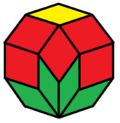With 10 rhombs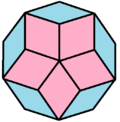With 10 rhombs

## Skew decagon

3 regular skew zig-zag decagons
{5}#{ } {5/2}#{ } {5/3}#{ }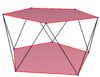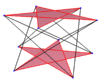A regular skew decagon is seen as zig-zagging edges of a pentagonal antiprism, a pentagrammic antiprism, and a pentagrammic crossed-antiprism.

A skew decagon is a skew polygon with 10 vertices and edges but not existing on the same plane. The interior of such an decagon is not generally defined. A skew zig-zag decagon has vertices alternating between two parallel planes.

A regular skew decagon is vertex-transitive with equal edge lengths. In 3-dimensions it will be a zig-zag skew decagon and can be seen in the vertices and side edges of a pentagonal antiprism, pentagrammic antiprism, and pentagrammic crossed-antiprism with the same D5d, [2+,10] symmetry, order 20.

These can also be seen in these 4 convex polyhedra with icosahedral symmetry. The polygons on the perimeter of these projections are regular skew decagons.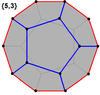Dodecahedron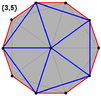Icosahedron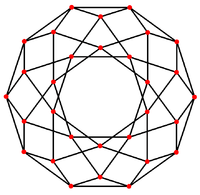Icosidodecahedron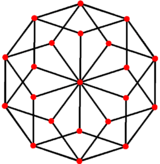Rhombic triacontahedron

### Petrie polygons

The regular skew decagon is the Petrie polygon for many higher-dimensional polytopes, shown in these orthogonal projections in various Coxeter planes: The number of sides in the Petrie polygon is equal to the Coxeter number, h, for each symmetry family.

A9 D6 B5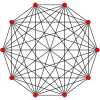9-simplex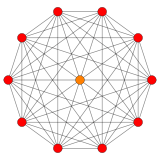411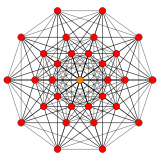131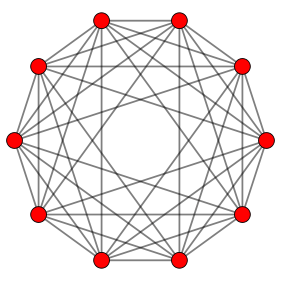5-orthoplex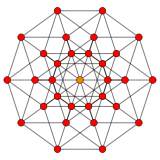5-cube

## References

1. Sidebotham, Thomas H. (2003), The A to Z of Mathematics: A Basic Guide, John Wiley & Sons, p. 146, ISBN 9780471461630.
2. Wenninger, Magnus J. (1974), Polyhedron Models, Cambridge University Press, p. 9, ISBN 9780521098595.
3. The elements of plane and spherical trigonometry, Society for Promoting Christian Knowledge, 1850, p. 59. Note that this source uses a as the edge length and gives the argument of the cotangent as an angle in degrees rather than in radians.
4. Ludlow, Henry H. (1904), Geometric Construction of the Regular Decagon and Pentagon Inscribed in a Circle, The Open Court Publishing Co..
5. Green, Henry (1861), Euclid's Plane Geometry, Books III–VI, Practically Applied, or Gradations in Euclid, Part II, London: Simpkin, Marshall,& CO., p. 116. Retrieved 10 February 2016.
6. (German) Köller, Jürgen (2005), Regelmäßiges Zehneck, → 3. Section "Formeln, Ist die Seite a gegeben ...". Retrieved 10 February 2016.
7. John H. Conway, Heidi Burgiel, Chaim Goodman-Strauss, (2008) The Symmetries of Things, ISBN 978-1-56881-220-5 (Chapter 20, Generalized Schaefli symbols, Types of symmetry of a polygon pp. 275-278)
8. Coxeter, Mathematical recreations and Essays, Thirteenth edition, p.141
9. Coxeter, Regular polytopes, 12.4 Petrie polygon, pp. 223-226.